# How to handle missing values of categorical variables in Python?

## How to handle missing values of categorical variables in Python?

Machine Learning is the field of study that gives computers the capability to learn without being explicitly programmed. Often we come across datasets in which some values are missing from the columns. This causes problems when we apply a machine learning model to the dataset. This increases the chances of error when we are training the machine learning model.

The dataset we are using is:

 `# import modules` `import` `pandas as pd` `import` `numpy as np` `# assign dataset` `df ``=` `pd.read_csv(``"train.csv"``, header``=``None``)` `df.head`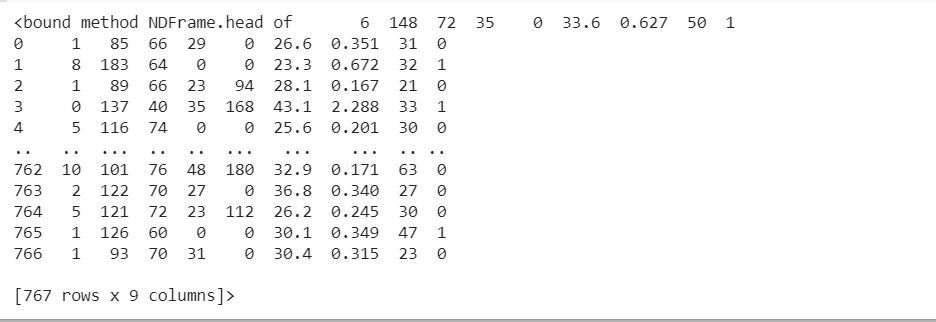Counting the missing data:

 `# counting number of values of all the columns` `cnt_missing ``=` `(df[[``1``, ``2``, ``3``, ``4``,` `                   ``5``, ``6``, ``7``, ``8``]] ``=``=` `0``).``sum``()` `print``(cnt_missing)`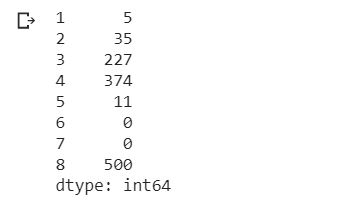We see that for 1,2,3,4,5 column the data is missing. Now we will replace all 0 values with NaN.

 `from` `numpy ``import` `nan` `df[[``1``, ``2``, ``3``, ``4``, ``5``]] ``=` `df[[``1``, ``2``, ``3``, ``4``, ``5``]].replace(``0``, nan)` `df.head(``10``)`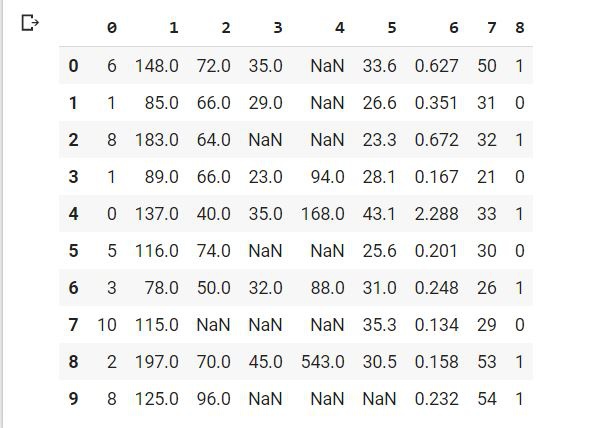Handling missing data is important, so we will remove this problem by following approaches:

#### Approach #1

The first method is to simply remove the rows having the missing data.

 `# printing initial shape` `print``(df.shape)` `df.dropna(inplace``=``True``)` `# final shape of the data with` `# missing rows removed` `print``(df.shape)`But in this, the problem that arises is that when we have small datasets and if we remove rows with missing data then the dataset becomes very small and the machine learning model will not give good results on a small dataset.

So to avoid this problem we have a second method. The next method is to input the missing values. We do this by either replacing the missing value with some random value or with the median/mean of the rest of the data.

#### Approach #2

We first impute missing values by the mean of the data.

 `# filling missing values` `# with mean column values` `df.fillna(df.mean(), inplace``=``True``)` `df.sample(``10``)`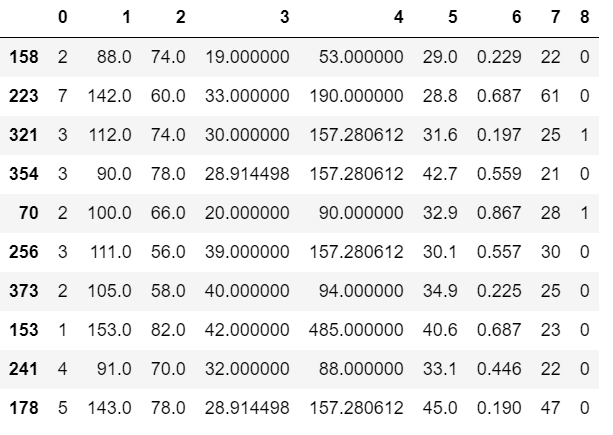We can also do this by using SimpleImputer class. SimpleImputer is a scikit-learn class which is helpful in handling the missing data in the predictive model dataset. It replaces the NaN values with a specified placeholder.It is implemented by the use of the SimpleImputer() method which takes the following arguments:

SimpleImputer(missing_values, strategy, fill_value)

• missing_values : The missing_values placeholder which has to be imputed. By default is NaN.
• strategy : The data which will replace the NaN values from the dataset. The strategy argument can take the values – ‘mean'(default), ‘median’, ‘most_frequent’ and ‘constant’.
• fill_value : The constant value to be given to the NaN data using the constant strategy.
 `# import modules` `from` `numpy ``import` `isnan` `from` `sklearn.impute ``import` `SimpleImputer` `value ``=` `df.values` `# defining the imputer` `imputer ``=` `SimpleImputer(missing_values``=``nan,` `                        ``strategy``=``'mean'``)` `# transform the dataset` `transformed_values ``=` `imputer.fit_transform(value)` `# count the number of NaN values in each column` `print``(``"Missing:"``, isnan(transformed_values).``sum``())`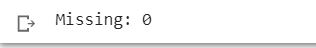#### Approach #3

We first impute missing values by the median of the data. Median is the middle value of a set of data. To determine the median value in a sequence of numbers, the numbers must first be arranged in ascending order.

 `# filling missing values` `# with mean column values` `df.fillna(df.median(), inplace``=``True``)` `df.head(``10``)`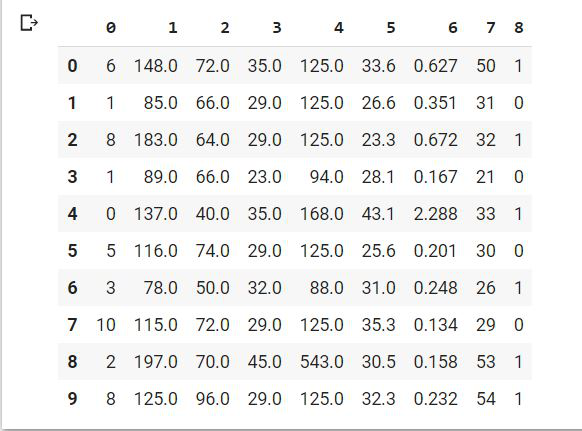We can also do this by using SimpleImputer class.

 `# import modules` `from` `numpy ``import` `isnan` `from` `sklearn.impute ``import` `SimpleImputer` `value ``=` `df.values` `# defining the imputer` `imputer ``=` `SimpleImputer(missing_values``=``nan,` `                        ``strategy``=``'median'``)` `# transform the dataset` `transformed_values ``=` `imputer.fit_transform(value)` `# count the number of NaN values in each column` `print``(``"Missing:"``, isnan(transformed_values).``sum``())`#### Approach #4

We first impute missing values by the mode of the data. The mode is the value that occurs most frequently in a set of observations. For example, {6, 3, 9, 6, 6, 5, 9, 3} the Mode is 6, as it occurs most often.

 `# filling missing values` `# with mean column values` `df.fillna(df.mode(), inplace``=``True``)` `df.sample(``10``)`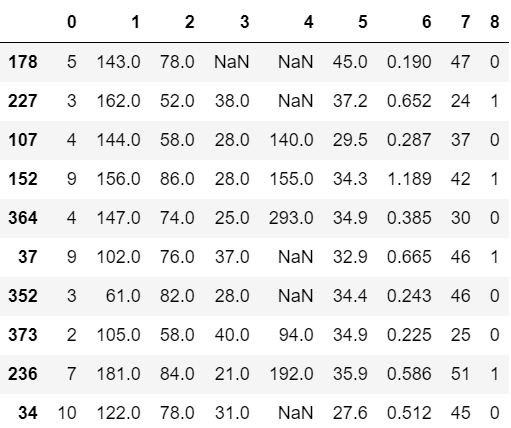We can also do this by using SimpleImputer class.

 `# import modules` `from` `numpy ``import` `isnan` `from` `sklearn.impute ``import` `SimpleImputer` `value ``=` `df.values` `# defining the imputer` `imputer ``=` `SimpleImputer(missing_values``=``nan,` `                        ``strategy``=``'most_frequent'``)` `# transform the dataset` `transformed_values ``=` `imputer.fit_transform(value)` `# count the number of NaN values in each column` `print``(``"Missing:"``, isnan(transformed_values).``sum``())`Last Updated on October 23, 2021 by admin

## Pandas Timestamp.replacePandas Timestamp.replace

Python | Pandas Timestamp.replace Python is a great language for doing data analysis, primarily because

## KDE Plot Visualization with Pandas and SeabornKDE Plot Visualization with Pandas and Seaborn

KDE Plot Visualization with Pandas and Seaborn KDE Plot described as Kernel Density Estimate is used for

## Pandas Series.isnull()Pandas Series.isnull()

Python Pandas Series.isnull() Pandas series is a One-dimensional ndarray with axis labels. The labels need

## Reverting from multiindex to single index dataframe in PandasReverting from multiindex to single index dataframe in Pandas

Reverting from multiindex to single index dataframe in Pandas In this article, we will be

## How to check whether the day is a weekday or not using Pandas in Python?How to check whether the day is a weekday or not using Pandas in Python?

Check whether the day is a weekday or not using Pandas in Python? Python is

## Python – Difference between Pandas.copy() and copying through variablesPython – Difference between Pandas.copy() and copying through variables

Python | Difference between Pandas.copy() and copying through variables Pandas .copy() method is used to create

## Pandas Profiling in PythonPandas Profiling in Python

Pandas Profiling in Python The pandas_profiling library in Python include a method named as ProfileReport()

## Pandas Groupby and SumPandas Groupby and Sum

Pandas Groupby and Sum Pandas is an open-source library that is built on top of NumPy library. It